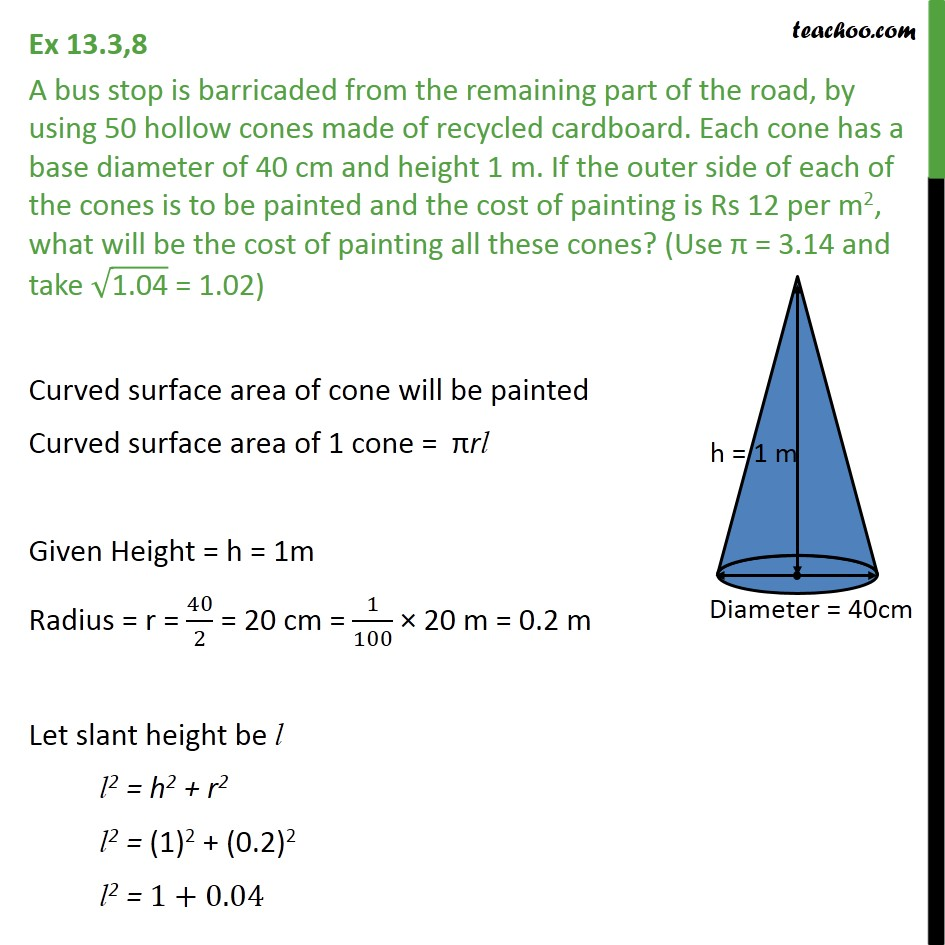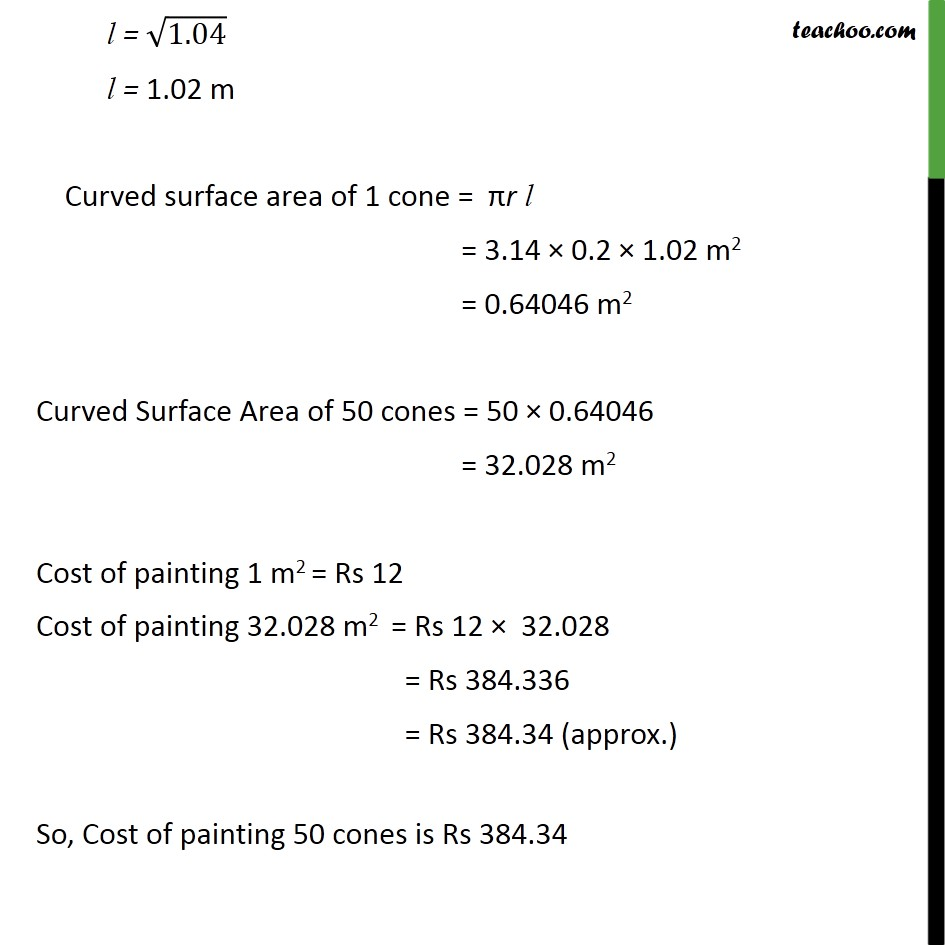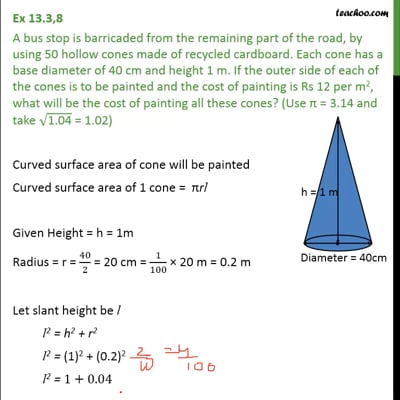Ex 13.3

Chapter 13 Class 9 Surface Areas and Volumes
Serial order wiseThis video is only available for Teachoo black users

Maths Crash Course - Live lectures + all videos + Real time Doubt solving!

### Transcript

Ex 13.3,8 A bus stop is barricaded from the remaining part of the road, by using 50 hollow cones made of recycled cardboard. Each cone has a base diameter of 40 cm and height 1 m. If the outer side of each of the cones is to be painted and the cost of painting is Rs 12 per m2, what will be the cost of painting all these cones? (Use π = 3.14 and take √("1.04" ) = 1.02) Curved surface area of cone will be painted Curved surface area of 1 cone = πrl Given Height = h = 1m Radius = r = 40/2 = 20 cm = 1/100 × 20 m = 0.2 m Let slant height be l l2 = h2 + r2 l2 = "(1)2 + (0.2)2" l2 = 1+0.04 l = √1.04 l = 1.02 m Curved surface area of 1 cone = πr l = 3.14 × 0.2 × 1.02 m2 = 0.64046 m2 Curved Surface Area of 50 cones = 50 × 0.64046 = 32.028 m2 Cost of painting 1 m2 = Rs 12 Cost of painting 32.028 m2 = Rs 12 × 32.028 = Rs 384.336 = Rs 384.34 (approx.) So, Cost of painting 50 cones is Rs 384.34